SEARCH HOMEMath Central Quandaries & QueriesQuestion from Dee, a parent: A guy goes 3.1m a second for 29 seconds in a golf cart. He then walks 1.5 meters a second. How long does he have to walk to get the average speed of 2.1m a second?Hi Dee.

Velocity (speed) is distance divided by time. We often writen this as: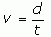(1)

We can solve this for d: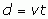(2)

So if we use the subscripts C and W to refer to the cart and walk parts and the subscript T to refer to the total, then we can write it this way: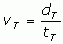(3)

Now since the total distance and time are just the sums of the two parts of the trip, we can replace dT and tT as shown below: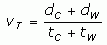(4)

Next we use equation (2) to replace dC and dW: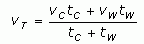(5)

It may look rather complicated, but you know all the values for these variables except tW. Solve for tW which is the time the man walks, substitute the values you know and you will answer your question.

Hope this helps,
Stephen La Rocque.Math Central is supported by the University of Regina and The Pacific Institute for the Mathematical Sciences.